## Recurrent Neural Networks

• Vanilla Neural Networks (i.e., feed-forward neural networks), input of fixed size goes through some hidden units and then go to output. We call it a one to one network.

• Recurrent Neural Networks RNN Models:

•• One to many
• Example: Image Captioning
• image ==> sequence of words
• Many to One
• Example: Sentiment Classification
• sequence of words ==> sentiment
• Many to many
• Example: Machine Translation
• seq of words in one language ==> seq of words in another language
• Example: Video classification on frame level
• RNNs can also work for Non-Sequence Data (One to One problems)

• So what is a recurrent neural network?

• Recurrent core cell that take an input x and that cell has an internal state that are updated each time it reads an input.

•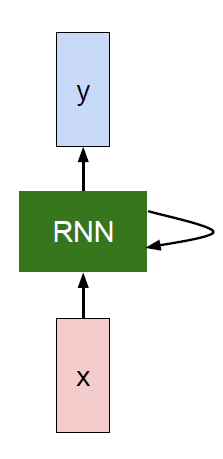• The RNN block should return a vector.

• We can process a sequence of vectors x by applying a recurrence formula at every time step:

• h[t] = fw (h[t-1], x[t])      # Where fw is some function with parameters W

• The same function and the same set of parameters are used at every time step.
• (Vanilla) Recurrent Neural Network:

• h[t] = tanh (W[h,h]*h[t-1] + W[x,h]*x[t])    # Then we save h[t]
y[t] = W[h,y]*h[t]

• This is the simplest example of a RNN.
• RNN works on a sequence of related data.

• Recurrent NN Computational graph:

•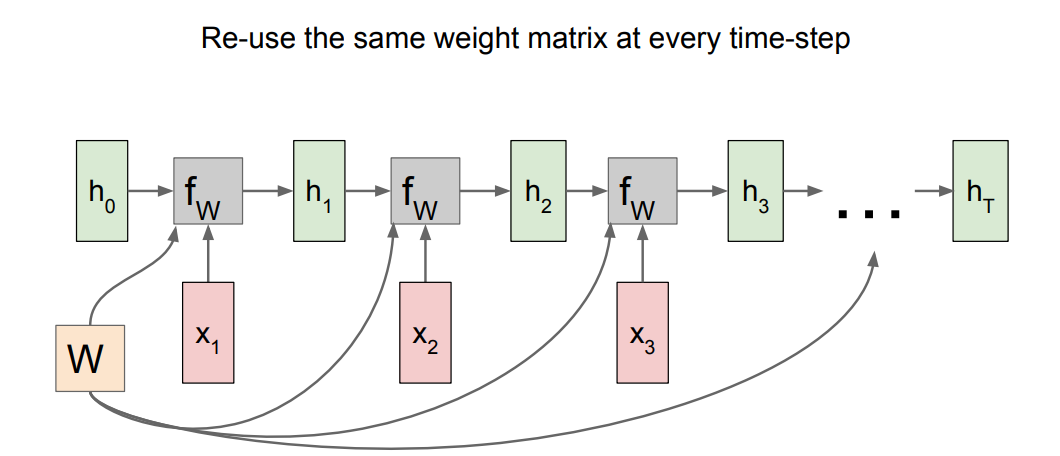• h0 are initialized to zero.
• Gradient of W is the sum of all the W gradients that has been calculated!
• A many to many graph:
•• Also the last is the sum of all losses and the weights of Y is one and is updated through summing all the gradients!
• A many to one graph:
•• A one to many graph:
•• sequence to sequence graph:
•• Encoder and decoder philosophy.
• Examples:

• Suppose we are building words using characters. We want a model to predict the next character of a sequence. Lets say that the characters are only [h, e, l, o] and the words are [hello]
• Training:
•• Only the third prediction here is true. The loss needs to be optimized.
• We can train the network by feeding the whole word(s).
• Testing time:
•• At test time we work with a character by character. The output character will be the next input with the other saved hidden activations.
• This link contains all the code but uses Truncated Backpropagation through time as we will discuss.
• Backpropagation through time Forward through entire sequence to compute loss, then backward through entire sequence to compute gradient.

• But if we choose the whole sequence it will be so slow and take so much memory and will never converge!
• So in practice people are doing “Truncated Backpropagation through time” as we go on we Run forward and backward through chunks of the sequence instead of whole sequence

• Then Carry hidden states forward in time forever, but only backpropagate for some smaller number of steps.
• Example on image captioning:

•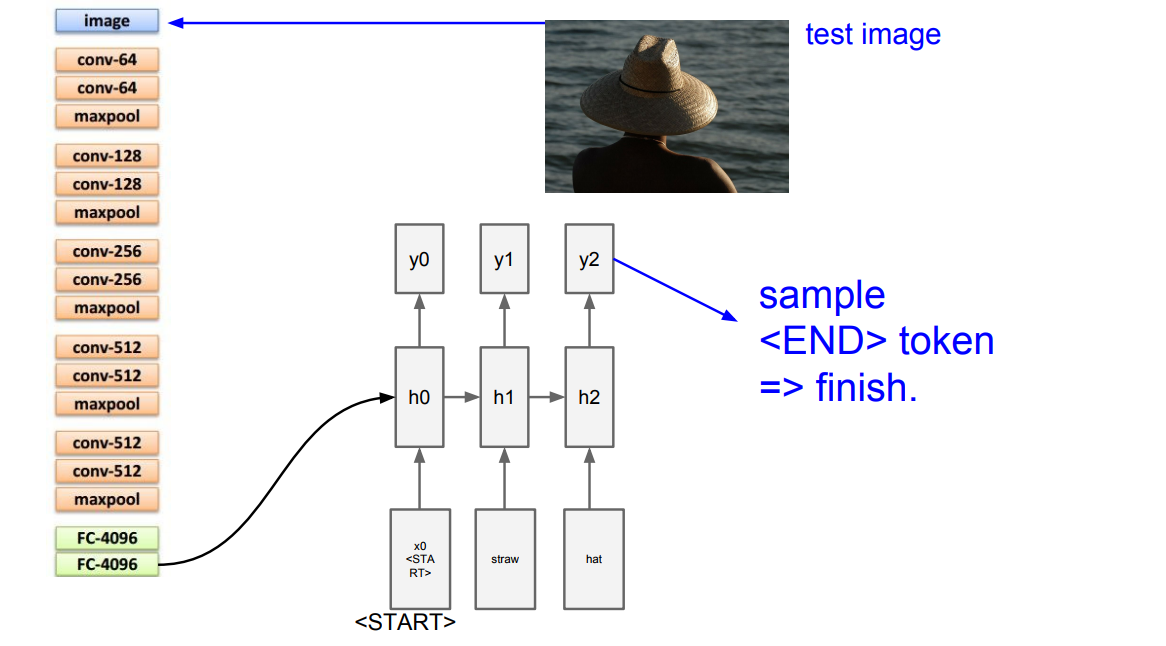• They use token to finish running.
• The biggest dataset for image captioning is Microsoft COCO.
• Image Captioning with Attention is a project in which when the RNN is generating captions, it looks at a specific part of the image not the whole image.

• Image Captioning with Attention technique is also used in “Visual Question Answering” problem
• Multilayer RNNs is generally using some layers as the hidden layer that are feed into again. LSTM is a multilayer RNNs.

• Backward flow of gradients in RNN can explode or vanish. Exploding is controlled with gradient clipping. Vanishing is controlled with additive interactions (LSTM)

• LSTM stands for Long Short Term Memory. It was designed to help the vanishing gradient problem on RNNs.

• It consists of:
• f: Forget gate, Whether to erase cell
• i: Input gate, whether to write to cell
• g: Gate gate (?), How much to write to cell
• o: Output gate, How much to reveal cell
•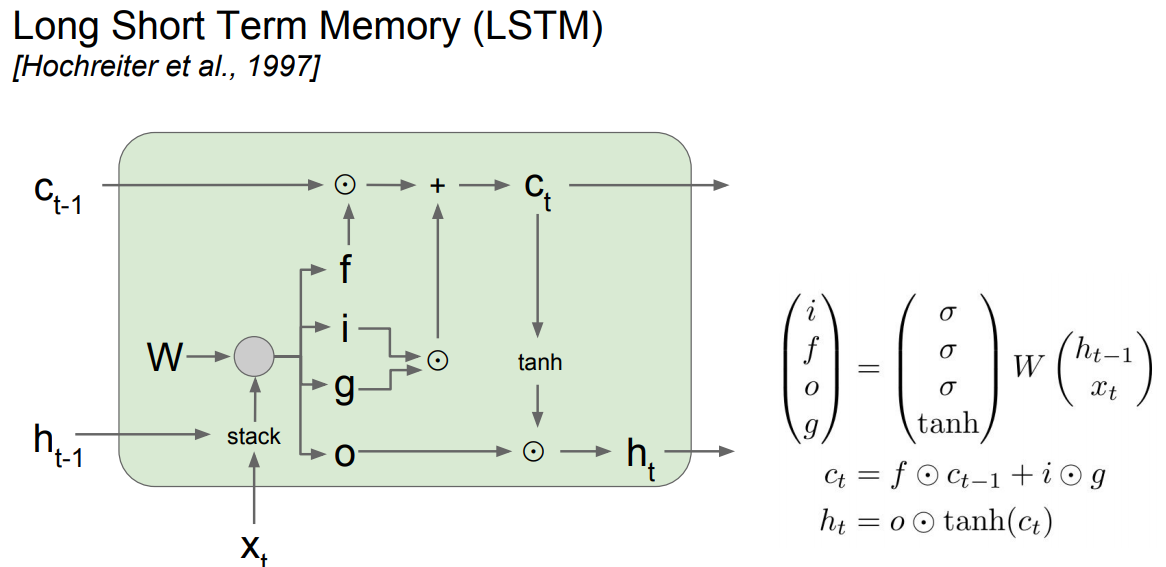•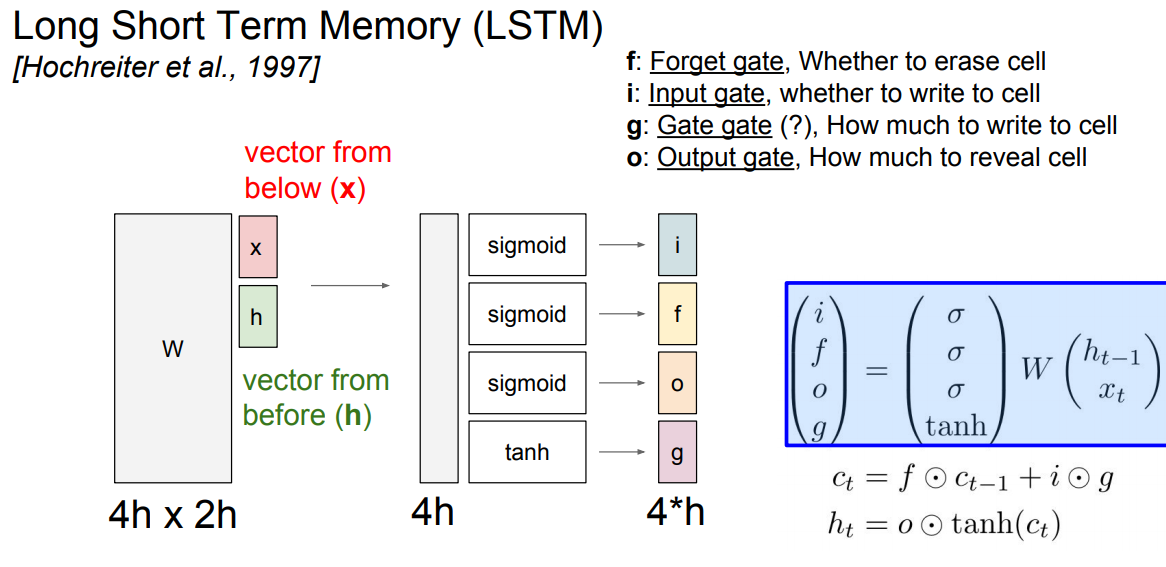• The LSTM gradients are easily computed like ResNet
• The LSTM is keeping data on the long or short memory as it trains means it can remember not just the things from last layer but layers.
• Highway networks is something between ResNet and LSTM that is still in research.

• Better/simpler architectures are a hot topic of current research

• Better understanding (both theoretical and empirical) is needed.

• RNN is used for problems that uses sequences of related inputs more. Like NLP and Speech recognition.

## Citation

If you found our work useful, please cite it as:

@article{Chadha2020RecurrentNeuralNetworks,
title   = {Recurrent Neural Networks},